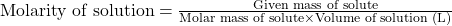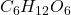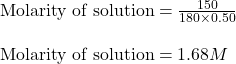## PLLLLEEAASEEE HELLPP!! What is the molarity of 150 g C6H12O6 per 0.50 L? A. 300 mol/L B. 75.0 mol/L C. 1

Question

PLLLLEEAASEEE HELLPP!!

What is the molarity of 150 g C6H12O6 per 0.50 L?

A. 300 mol/L
B. 75.0 mol/L
C. 1.68 mol/L
D. 0.833 mol/L

in progress 0
6 months 2021-07-21T13:03:29+00:00 1 Answers 4 views 0

1. Answer: The correct option is C) 1.68 mol/L

Explanation:

Molarity is defined as the amount of solute expressed in the number of moles present per liter of solution. The units of molarity are mol/L. The formula used to calculate molarity:…..(1)

Given values:

Given mass of= 150 g

Molar mass of= 180 g/mol

Volume of the solution = 0.50 L

Putting values in equation 1, we get:Hence, the correct option is C) 1.68 mol/L